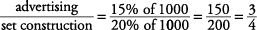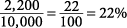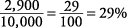## Circle Graphs or Pie Charts

A circle graph, or pie chart, shows the relationship between the whole circle (100%) and the various slices that represent portions of that 100%. The larger the slice, the higher the percentage.
##### Example 1

Based on the circle graph shown in Figure 1,

1. If Smithville Community Theater has \$1,000 to spend this month, how much will be spent on set construction?

2. What is the ratio of the amount of money spent on advertising to the amount of money spent on set construction?

1. The theater spends 20% of its money on set construction. 20% of \$1,000 is \$200, so \$200 will be spent on set construction.

2. To answer this question, you must use the information in the graph to make a ratio.Notice thatsimplifies to.

Figure 1. Circle graph.##### Example 2

Based on the circle graph shown in Figure 2,

1. If the Bell Canyon PTA spends the same percentage on dances every year, how much will they spend on dances in a year in which their total amount spent is \$15,000?

2. The amount of money spent on field trips in 1995 was approximately what percent of the total amount spent?

1. To answer this question, you must find a percent and then apply this percent to a new total. In 1995, the PTA spent \$2,200 on dances. This can be calculated to be 22% of the total spent in 1995 by the following method.Now, multiplying 22% times the new total amount spent of \$15,000 gives the right answer.You could use another common‐sense method. If \$2,200 out of \$10,000 is spent for dances, \$1,100 out of every \$5,000 is spent for dances. Since \$15,000 is 3 × \$5,000, 3 × \$1,100 would be \$3,300.

2. By carefully reading the information in the graph, you find that \$2,900 was spent on field trips. The information describing the graph explains that the total expenditures were \$10,000. The approximate percentage would be worked out as follows.Figure 2. Circle graph of 1995 budget of the Bell Canyon PTA.